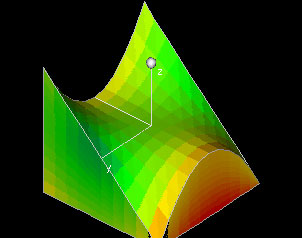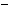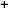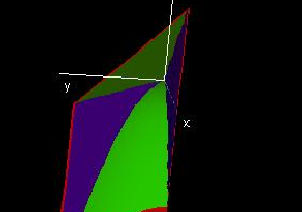Labware - MA35 Multivariable Calculus - Three Variable Calculus
 MA35 Labs 3 » Three Variable Calculus Contents3.4 Integration 3.4.3 Total Mass of Regions between Function Graphs 3.4.5 Change of Variables 3.4.6 Center of Mass 3.4.7 Moment of Intertia Search

Total Mass of Regions between Function Graphs

Text

As was true with simple regions, in order to find the total mass of a region between two function graphs, we must integrate the density function times some tiny volume dV over the given region. The difference here is in the limits of integration. x and y will still begin and end at constants. The lower and upper limits of z, however, will be functions of x and y.

DemosThis demo shows a density function f(x,y,z) defined over a region in three-space, and it computes the total mass contained in the region. The region is bounded by the six surfaces x=a, x=b, y=c(x), y=d(x) q(x,y) and p(x,y), so you can change the region by entering different values for a and b, by entering different curves c(x), d(x) or by entering different surfaces p(x,y) and q(x,y).A region in 3-spaceThis demo can serve as a tool for integrating over regions in three-space, more specifically it will help you to get the boundaries of triple integrals right - this can be rather tricky if you are changing the order of integration. You can use the demo to visualize any three-dimensional region that is bounded by a particular kind of surface. (You will see when you open the demo.)Exercises

• 1. How can you modify the problem of finding the mass of a region between two function graphs so that you only need to consider one function graph?
• 2. Find two functions of x, y, and z which enclose a region whose volume is finite (i.e. they intersect to form a closed surface).